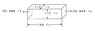# Longitudinal elongation

• misty777
In summary, the conversation is about a person seeking help in calculating the longitudinal elongation of a steel bar. They are unsure of where to start and ask for guidance on which formula to use. The conversation also mentions the importance of showing work and using relevant equations when posting homework questions. Eventually, the person is able to calculate the elongation by using the formula for stress and strain, as well as Hooke's law. The final answer is 8 inches, which is confirmed by another person in the conversation.

#### misty777

Hi. I am hoping someone can be of assistance in helping me solve a problem.

I am trying to calculate the longitudinal elongation of the steel bar shown in the attachment. I don't know where to start, so if anyone could point me in the right direction such as which formula I should use I would really appreciate it.

#### Attachments

•problem6.jpg
6 KB · Views: 649
Welcome to the PF, misty777. Keep in mind that homework and coursework problems are to be posted in the Homework Help forums area, and not here in the general forums. And when you post homework problems, you should use the Homework Help Template that is provided when you start a thread in those forums.

I'll leave this question here for now, but it may get moved to one of the Homework Help forums at some point (and your other thread here with a similar question may be moved as well).

With homework and coursework questions, you are required to show us your work and the relevant equations, in order for us to help you better. So, please define stress and strain for us. What modulus relates stress and strain? How can you use those concepts to solve this problem?

Thank you for the warm welcome.

I think the fomula needed to find elongation is:

Axial tension strain equals change in length divided by original length

I know that the original length is 100 ft, but I still need to find the stain in order to complete the problem.

I think this requires using the modules of elasticity formula to find the stain

Modulus of elasticity equals stress divided by stain

I know the modulus of elasticity of steel is 30,000,000 psi, but I do not know the stress.

I think stress can be found by dividing the load (50,000 lb) by the area (2400 sq in).

When I work this out it doesn't come out right. I know the answer to this problem is 8in. I've even tried working it backwards, but I am either missing a step or calculating something wrong. I would really like to understand the steps to work this problem.

Thank you.

Well I don't know how to guide you more here, so I'll just say it, but really try to think more of the physical problem rather than formulas next time. You force is acting on the cross-section of the beam, ONLY that area is needed to calculate the stress by: stress=force/area, then you use Hooke's law for elongation as you described and mind the units.

Thank you for your help. I think I am understanding now.

Stress=force/area
therefore stress = 50,000/.25
so the stress is 200,000 psi

then using Hooke's law..
strain equals stress divided by modulus of elasticity
therefore strain equals 200,000/30,000,000
so strain is .0066667

then to find the amount of elongation..
Axial tension strain equals change in length divided by original length
therefore .0066667 = x/1200in
x=8
so the change in length is 8 in

I know the answer is supposed to be 8 in, have I done the process correctly this time?

Holy mackerel. 50 kips on a .5 in square piece of steel? Nice to see they're keeping the problems in the realm of reality.

How about just calculating the deflection directly?

Does

$$\delta = \frac{PL}{AE}$$

look familiar?

Last edited:
Thank you for the tip FredGarvin. I wasn't aware I could do it that way.